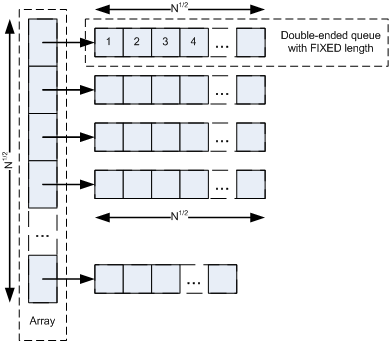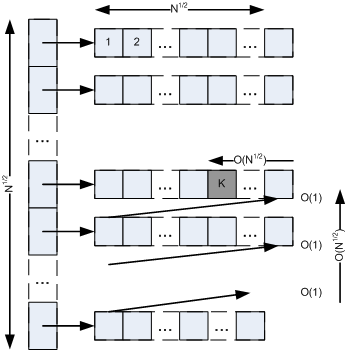# GitHub – igushev/IgushArray

## Overview

There are two basic data structures: array and list. Array is a random
access data structure but with an expensive line-time insert/erase
operation. On the contrary, list is a sequential access data structure
but it has fast constant-time insert/erase operation. In this paper I’ll
describe a random access data structure named modestly IgushArray which
like an array has fast constant-time access operation, but insert/erase
operation takes only O (N^1/2) time. The structure can be considered as
“fast array” or “array with fast insert operation”. Comparison to other
structures can be seen in the table below.

Operation Array IgushArray List
Access O (1) O (1) O (N)
Insert O (N) O (N^1/2) O (1)
Erase O (N) O (N^1/2) O (1)
Push Back O (1)* O (1)* O (1)
Push Front O (N) O (N^1/2) O (1)

## Motivation

Motivation was the need for an array which can be kept sorted and one could
quickly access k-th max element and at the same time quickly insert or delete
an element.

## Implementations

• This implementation written in C++. The IgushArray class fully implements
std::vector interface
, so it is very easy to replace std::vector in code;

• C# – cser/IgushArray;

## Structure

IgushArray is an array of pointers with approximate size of N^1/2 and
each element points to a double-ended queue with approximate size of
N^1/2. All DEQs have the same size except last one (last one can
have less than N^1/2 size). See picture below.

The size of the array can be changed during work with the structure; the
size of DEQs is never changed. But after some work with a structure
it can be done total reconstruction to adjust array and DEQs size to
approximately N^1/2. It is also highly recommended to know the
approximate size of a future structure during creation to initially
calculate optimal sizes. Otherwise the size of DEQ would 1 and
insert/erase time would degenerate to linear.

A DEQ can be implemented using array (set of arrays) or list. In first
case it provides constant-time access, but linear-time insert/erase, in
second case it provides linear-time access, but constant-time
insert/erase. It is very important that in IgushArray s DEQ should be
implemented using first approach. Also in IgushArray the size of DEQs is
know during creation and never changed, so this fact can be used and a
DEQ can be implemented using only one array which would improve and
simplify the implementation.

So, array and DEQ, used in IgushArray implementation, itself are random
access data structures.## Access

To access a random element by its index in IgushArray, it is needed to
calculate index in pointers array by K / deq_size operation ( O (1)
), than obtain the pointer ( O (1) ), calculate index in DEQ by K %
deq_size
operation ( O (1) ) and obtain the element in DEQ. See
picture below. It can be seen, that access operation has constant-time
complexity.## Insertion

To insert a new element into IgushArray, it is needed to insert the
element into particular position in particular DEQ ( O (N^1/2) ) and
“move” the elements in this and the next DEQs down. “Moving” element
down means that it’s needed to pop an element from the end of a DEQ and
push it to the beginning to the next one. Each moving has O (1)
complexity. It is needed to “move” down less than N^1/2 elements ( O
(N^1/2) ). See picture below. It can be seen, that total operation has
O (N^1/2) complexity.

An insertion may require a push back to the end of the pointers array
and creation of a new DEQ (in case of the last DEQ is full). The
operation may take O (N^1/2) time and wouldn’t damage total
performance.## Erasing

To erase an element in IgushArray, it is needed to erase the element in
particular DEQ ( O (N^1/2) ) and “move” the elements in this and the
next DEQs up. “Moving” element up means that it’s needed to pop an
element from the beginning of a DEQ and push it to the end to the
previous one. Each moving has O (1) complexity. It is needed to “move”
up less than N^1/2 elements ( O (N^1/2) ). See picture below. It can
be seen, that total operation has O (N^1/2) complexity.

An erasing can require a destruction of last DEQ (in case of the last
DEQ has one element).## Generalization

It can be noticed that array which is used in DEQ implementation can be
IgushArray by itself and so on. In this case the complexity of access
operation would be O (k) where k is degree; the complexity of
insert/erase operation would be O (N1/k). In simple case described in
this paper k is 2.

The package performance test results can be seen below. The tests were
done using computer with next configuration: Inter Core 2 Duo 1,83MHz,
2GB, Ubuntu, GCC version 4.5.2. The results are in milliseconds. Every
operation was performed 1000 times.

## Access by Index

Below there are the results of calculating the sum of a structure.

Count IgushArray std::vector Result
1 000 80 10 Slower: 8
10 000 840 110 Slower: 7.6
100 000 8220 1130 Slower: 7.3
1 000 000 89340 15720 Slower: 5.7
10 000 000 903520 130200 Slower: 6.9

It can be seen that the time of sum grows linear as count of elements.
It means that the both structures have constant-time access operation.

## Access by Iterator

Below there are the results of calculating the sum of a structure.

Count IgushArray std::vector Result
1 000 170 40 Slower: 4.2
10 000 1700 340 Slower: 5
100 000 16910 3420 Slower: 4.9
1 000 000 167600 34310 Slower: 4.9
10 000 000 1686220 343090 Slower: 4.9

It can be seen that the time of sum grows linear as the count of
elements.

The worse access time of IgushArray is due to IgushArray generally is
more complicated structure than array.

## Insertion

Below there are results of inserting one element in the middle.

Count IgushArray std::vector Result
1 000 10 10 Same time
10 000 10 30 Faster: 3
100 000 60 360 Faster: 6
1 000 000 80 3580 Faster: 45
10 000 000 550 36940 Faster: 67

It can be seen that the time of std::vector insert operation grows
linear as the count of elements, but the time of IgushArray insert
operation grows like N^1/2.

## Erasing

Below there are results of erasing one element from the middle.

Count IgushArray std::vector Result
1 000 10 10 Same time
10 000 40 10 Slower: 4
100 000 60 300 Faster: 5
1 000 000 200 3270 Faster: 16
10 000 000 590 31310 Faster: 53

It can be seen that the time of std::vector erase operation grows linear
as the count of elements, but the time of IgushArray erase operation
grows like N^1/2.

## Structure

This implementation written in C++. The IgushArray class fully implements
std::vector interface
, so it is very easy to replace std::vector in code.

It is highly recommended to know the approximate size of a future
structure and transfer it to the constructor or immediately after
creation to reserve() function to initially calculate optimal sizes.
Otherwise, the size of DEQ would 1 and insert/erase time would
degenerate to linear.

The implementation also provides FixedDeque class. The class is a simple
double-ended queue which uses only one array in its implementation and
requires its size during creation (in constructor). It does not fully
implements std::deque interface and provides only functions needed by
IgushArray implementation.

## Limitations

Regardless of the IgushArray class implements std::vector class, there
are cases when std::vector is used as a buffer. In these cases,
std::vector cannot be replaced, because in IgushArray structure elements
are not stored sequentially in memory. Also in these cases insert and
erase time is not an issue.

IgushArray class fully provides iterator mechanism, but this mechanism
does not guarantee an iterator consistence after modifying operations
such as insert/erase, push back/pop back and so on.

## Test Packs

The package has also two stability test packs and performance test pack.
Stability test pack tests almost all IgushArray interface functions and
compares results it with std::vector as a baseline. There is same pack
for FixedDeque.

Performance test pack compares the results of main IgushArray functions
(access, insert/erase) performance with std::vector performance. Main
dependences can be seen using this pack (see below).

## Port to Other Languages

https://github.com/igushev/IgushArray